# Calculating the Interest Rate (i)

Now we will show how to find the interest rate (i) for discounting the future amount in a present value (PV) calculation. To do this, we need to know the three other components in the PV calculation: present value amount (PV), future amount (FV), and the length of time before the future amount is received (n).

### Exercise #8

What is the annual interest rate necessary to discount a future amount of \$10,000 to a present value of \$7,300 over a four-year period, assuming the interest is compounded annually?

The following timeline depicts the information we know, along with the unknown component (i):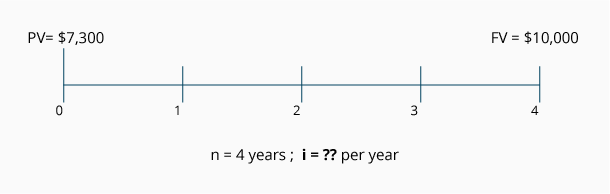Calculation Using a Present Value of 1 Table
As the timeline indicates, we know the future value is \$10,000 and the present value is \$7,300. The number of years (n) is four. The unknown component is the annual interest rate (i), which is compounded annually. In equation form, Exercise #8 looks like this: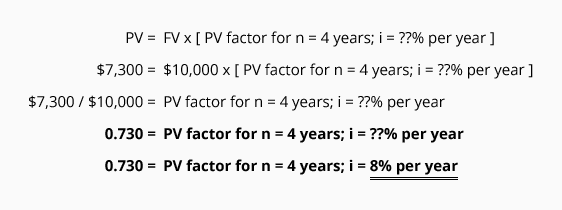Our equation tells us that the PV factor is 0.730. Since the number of periods is four, we look at the PV of 1 Table in the row where n = 4 and find the amount closest to 0.730; this would be 0.735. We see it is located in the column where i = 8%. This tells us that the missing component, the annual interest rate, is approximately 8% compounded annually.

### Exercise #9

What is the annual interest rate necessary to discount a future amount of \$100,000 to a present value of \$67,000 over a five-year period, assuming the interest is compounded quarterly?

The following timeline depicts the information we know, along with the unknown component (i):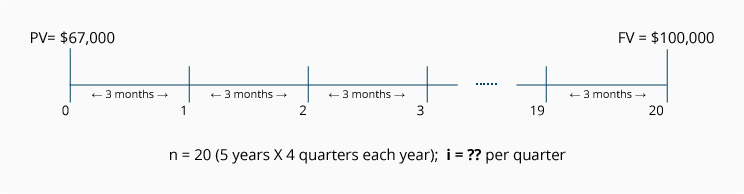Calculation Using a PV of 1 Table
As the timeline indicates, we know the future value is \$100,000 and the present value is \$67,000. The number of periods (n) is 20 quarters (5 years x 4 quarters per year). The unknown component is the interest rate (i), which will be expressed as a quarterly rate. In equation form, Exercise #9 looks like this: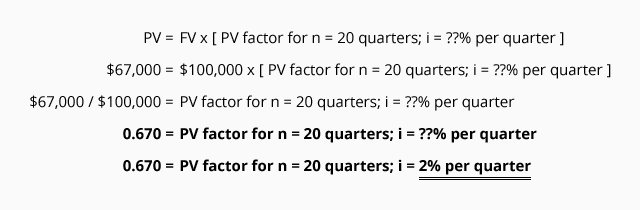Our equation tells us that the PV factor is 0.670. Since the number of periods is 20 quarters, we look at the PV of 1 Table in the row where n = 20 and we find the amount closest to 0.670; this would be 0.673. We see it is located in the column where i = 2%. This tells us that the missing component, the interest rate is approximately 2% per quarter. However, the exercise asked for the annual interest rate, compounded quarterly. The annual interest rate is approximately 8% (the quarterly interest rate x four quarters).

### Exercise #10

A future amount of \$1,000 will be due in exactly two years. The obligation can be settled today for \$790. What is the annual interest rate involved in this arrangement if interest is compounded monthly?

The following timeline depicts the information we know, along with the unknown component (i):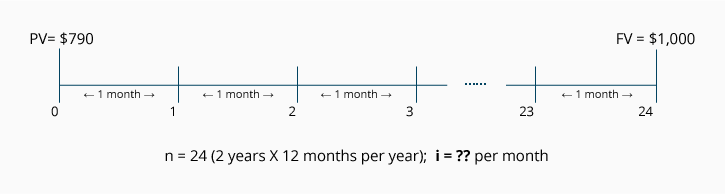Calculation Using a PV of 1 Table
As the timeline indicates, we know the future value is \$1,000 and the present value is \$790. Since the interest is compounded monthly, the number of time periods (n) is 24 (2 years x 12 months per year). The unknown component is the monthly interest rate (i).

In equation form, Exercise #10 looks like this: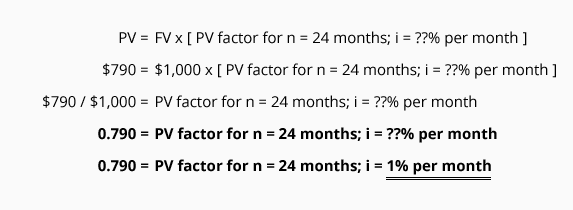Our equation tells us that the PV factor is 0.790. Since the number of periods is 24 months, we look at the PV of 1 Table in the row where n = 24 and find the amount closest to 0.790; this would be 0.788. We see it is located in the column where i = 1%.

This tells us that the missing component, the interest rate (i), is approximately 1% per month. However, the exercise asked for the annual interest rate, compounded monthly. The annual interest rate is approximately 12% (the approximate monthly interest rate x 12 months).